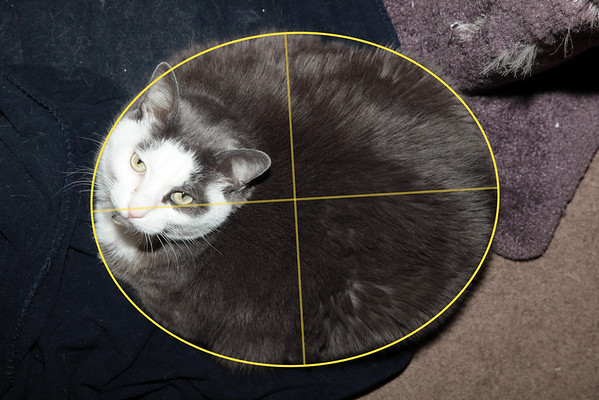I looked at my cat Jack last week and noticed a curious thing. When he sits in a loaf he is quite the ellipse.Of course this leads to only one possible next step: computing his eccentricity.

Let’s take a step back. A circle is just a degenerate ellipse. A circle just has an eccentricity of zero. Similarly, a straight line has an eccentricity of one. The way you compute eccentricity is:

`e = Sqrt(1 - (b/a)^2)`

a is the length of the major axis (the long one) and b is the length of the minor axis.

In this case (this is a unitless measurement) a = 54.0238 and b is 43.2835. (Technically this is in inches in Adobe Illustrator, but the pixels per inch doesn’t really correspond to anything. The units would cancel out anyway)

Therefore, Jack has an eccentricity of 0.598406.

This is if he were just like the picture: two dimensional. Of course he is more of an oblate spheroid, but I’m too lazy to do that math now.

Eccentric Jack Workbook

[smugmug url=”http://photos.vec.com/hack/feed.mg?Type=gallery&Data=15090417_KuXwg&format=rss200″ imagecount=”100″ start=”1″ num=”100″ thumbsize=”Th” link=”lightbox” captions=”false” sort=”true” window=”true” smugmug=”true” size=”L”]